## DFA to Regular Expression-

The two popular methods for converting a given DFA to its regular expression are-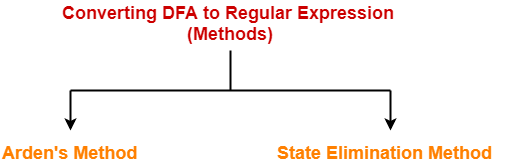1. Arden’s Method
2. State Elimination Method

## Arden’s Theorem-

Arden’s Theorem is popularly used to convert a given DFA to its regular expression.

It states that-

Let P and Q be two regular expressions over ∑.

If P does not contain a null string ∈, then-

R = Q + RP has a unique solution i.e. R = QP*

## Conditions-

To use Arden’s Theorem, following conditions must be satisfied-

• The transition diagram must not have any ∈ transitions.
• There must be only a single initial state.

## Steps-

To convert a given DFA to its regular expression using Arden’s Theorem, following steps are followed-

### Step-01:

• Form a equation for each state considering the transitions which comes towards that state.
• Add ‘∈’ in the equation of initial state.

### Step-02:

Bring final state in the form R = Q + RP to get the required regular expression.

## Important Notes-

### Note-01:

Arden’s Theorem can be used to find a regular expression for both DFA and NFA.

### Note-02:

If there exists multiple final states, then-

• Write a regular expression for each final state separately.
• Add all the regular expressions to get the final regular expression.

## Problem-01:

Find regular expression for the following DFA using Arden’s Theorem-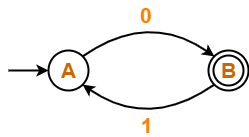## Solution-

### Step-01:

Form a equation for each state-

• A = ∈ + B.1     ……(1)
• B = A.0           ……(2)

### Step-02:

Bring final state in the form R = Q + RP.

Using (1) in (2), we get-

B = (∈ + B.1).0

B = ∈.0 + B.1.0

B = 0 + B.(1.0)          ……(3)

Using Arden’s Theorem in (3), we get-

B = 0.(1.0)*

Thus, Regular Expression for the given DFA = 0(10)*

## Problem-02:

Find regular expression for the following DFA using Arden’s Theorem-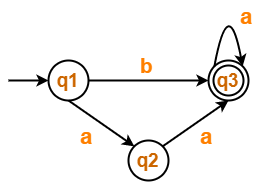## Solution-

### Step-01:

Form a equation for each state-

• q1 = ∈                                        ……(1)
• q2 = q1.a                                   ……(2)
• q3 = q1.b + q2.a + q3.a            …….(3)

### Step-02:

Bring final state in the form R = Q + RP.

Using (1) in (2), we get-

q2 = ∈.a

q2 = a              …….(4)

Using (1) and (4) in (3), we get-

q3 = q1.b + q2.a + q3.a

q3 = ∈.b + a.a + q3.a

q3 = (b + a.a) + q3.a        …….(5)

Using Arden’s Theorem in (5), we get-

q3 = (b + a.a)a*

Thus, Regular Expression for the given DFA = (b + aa)a*

## Problem-03:

Find regular expression for the following DFA using Arden’s Theorem-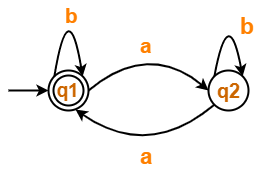## Solution-

### Step-01:

Form a equation for each state-

• q1 = ∈ + q1.b + q2.a                 ……(1)
• q2 = q1.a + q2.b                       ……(2)

### Step-02:

Bring final state in the form R = Q + RP.

Using Arden’s Theorem in (2), we get-

q2 = q1.a.b*             …….(3)

Using (3) in (1), we get-

q1 = ∈ + q1.b + q1.a.b*.a

q1 = ∈ + q1.(b + a.b*.a)          …….(4)

Using Arden’s Theorem in (4), we get-

q1 = ∈.(b + a.b*.a)*

q1 = (b + a.b*.a)*

Thus, Regular Expression for the given DFA = (b + a.b*.a)*

## Problem-04:

Find regular expression for the following DFA using Arden’s Theorem-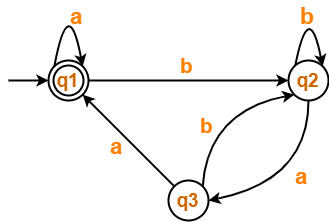## Solution-

### Step-01:

Form a equation for each state-

• q1 = ∈ + q1.a + q3.a                 ……(1)
• q2 = q1.b + q2.b + q3.b            ……(2)
• q3 = q2.a                                  …….(3)

### Step-02:

Bring final state in the form R = Q + RP.

Using (3) in (2), we get-

q2 = q1.b + q2.b + q2.a.b

q2 = q1.b + q2.(b + a.b)          …….(4)

Using Arden’s Theorem in (4), we get-

q2 = q1.b.(b + a.b)*    …….(5)

Using (5) in (3), we get-

q3 = q1.b.(b + a.b)*.a       …….(6)

Using (6) in (1), we get-

q1 = ∈ + q1.a + q1.b.(b + a.b)*.a.a

q1 = ∈ + q1.(a + b.(b + a.b)*.a.a)        …….(7)

Using Arden’s Theorem in (7), we get-

q1 = ∈.(a + b.(b + a.b)*.a.a)*

q1 = (a + b.(b + a.b)*.a.a)*

Thus, Regular Expression for the given DFA = (a + b(b + ab)*aa)*

To gain better understanding about Arden’s Theorem,

Watch this Video Lecture

Next Article- Non-Deterministic Finite Automata

Get more notes and other study material of Theory of Automata and Computation.

Watch video lectures by visiting our YouTube channel LearnVidFun.

## DFA to Regular Expression-

The two popular methods for converting a DFA to its regular expression are-1. Arden’s Method
2. State Elimination Method

## State Elimination Method-

This method involves the following steps in finding the regular expression for any given DFA-

### Thumb Rule

The initial state of the DFA must not have any incoming edge.

• If there exists any incoming edge to the initial state, then create a new initial state having no incoming edge to it.

### Example-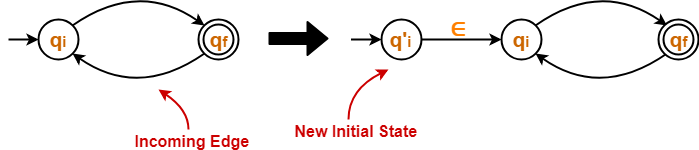### Thumb Rule

There must exist only one final state in the DFA.

• If there exists multiple final states in the DFA, then convert all the final states into non-final states and create a new single final state.

### Example-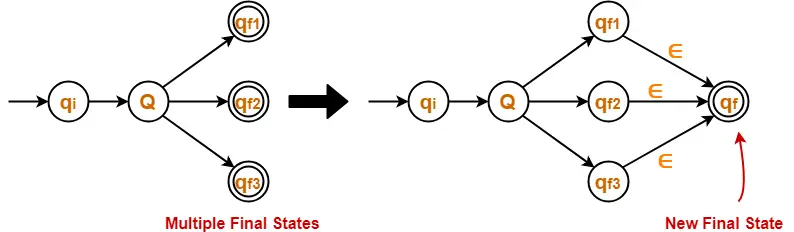### Thumb Rule

The final state of the DFA must not have any outgoing edge.

• If there exists any outgoing edge from the final state, then create a new final state having no outgoing edge from it.

### Example-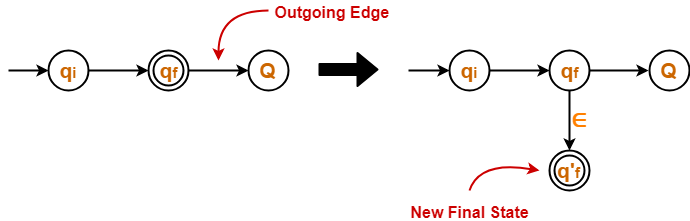### Step-04:

• Eliminate all the intermediate states one by one.
• These states may be eliminated in any order.

In the end,

• Only an initial state going to the final state will be left.
• The cost of this transition is the required regular expression.

### NOTE

The state elimination method can be applied to any finite automata.

(NFA, ∈-NFA, DFA etc)

## Problem-01:

Find regular expression for the following DFA-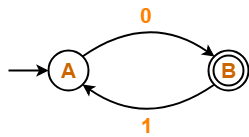## Solution-

### Step-01:

• Initial state A has an incoming edge.
• So, we create a new initial state qi.

The resulting DFA is-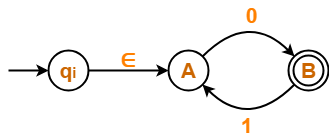### Step-02:

• Final state B has an outgoing edge.
• So, we create a new final state qf.

The resulting DFA is-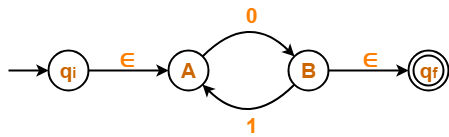### Step-03:

Now, we start eliminating the intermediate states.

First, let us eliminate state A.

• There is a path going from state qi to state B via state A.
• So, after eliminating state A, we put a direct path from state qi to state B having cost ∈.0 = 0
• There is a loop on state B using state A.
• So, after eliminating state A, we put a direct loop on state B having cost 1.0 = 10.

Eliminating state A, we get-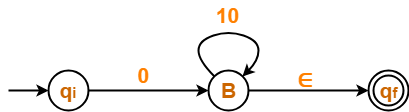### Step-04:

Now, let us eliminate state B.

• There is a path going from state qi to state qf via state B.
• So, after eliminating state B, we put a direct path from state qi to state qf having cost 0.(10)*.∈ = 0(10)*

Eliminating state B, we get-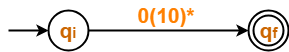From here,

 Regular Expression = 0(10)*

### NOTE-

In the above question,

• If we first eliminate state B and then state A, then regular expression would be = (01)*0.
• This is also the same and correct.

## Problem-02:

Find regular expression for the following DFA-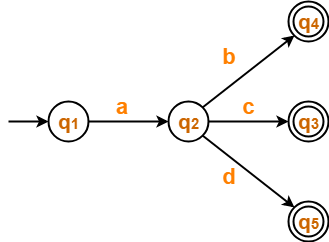## Solution-

### Step-01:

• There exist multiple final states.
• So, we convert them into a single final state.

The resulting DFA is-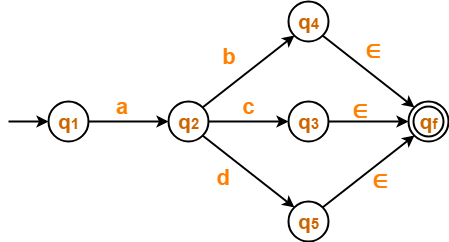### Step-02:

Now, we start eliminating the intermediate states.

First, let us eliminate state q4.

• There is a path going from state q2 to state qf via state q4.
• So, after eliminating state q4 , we put a direct path from state q2 to state qf having cost b.∈ = b.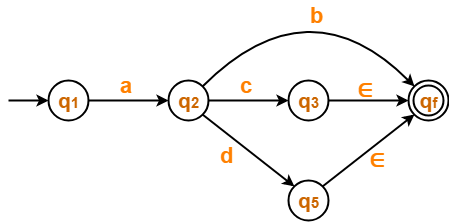### Step-03:

Now, let us eliminate state q3.

• There is a path going from state q2 to state qf via state q3.
• So, after eliminating state q3 , we put a direct path from state q2 to state qf having cost c.∈ = c.### Step-04:

Now, let us eliminate state q5.

• There is a path going from state q2 to state qf via state q5.
• So, after eliminating state q5 , we put a direct path from state q2 to state qf having cost d.∈ = d.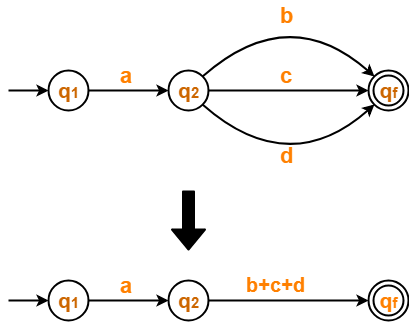### Step-05:

Now, let us eliminate state q2.

• There is a path going from state q1 to state qf via state q2.
• So, after eliminating state q2 , we put a direct path from state q1 to state qf having cost a.(b+c+d).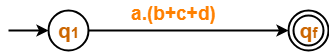From here,

 Regular Expression = a(b+c+d)

## Problem-03:

Find regular expression for the following DFA-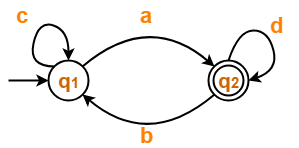## Solution-

### Step-01:

• Initial state q1 has an incoming edge.
• So, we create a new initial state qi.

The resulting DFA is-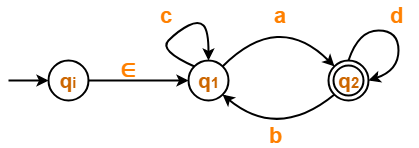### Step-02:

• Final state q2 has an outgoing edge.
• So, we create a new final state qf.

The resulting DFA is-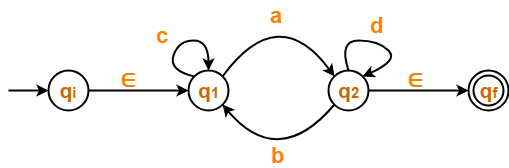### Step-03:

Now, we start eliminating the intermediate states.

First, let us eliminate state q1.

• There is a path going from state qi to state q2 via state q1 .
• So, after eliminating state q1, we put a direct path from state qi to state q2 having cost ∈.c*.a = c*a
• There is a loop on state q2 using state q1 .
• So, after eliminating state q1 , we put a direct loop on state q2 having cost b.c*.a = bc*a

Eliminating state q1, we get-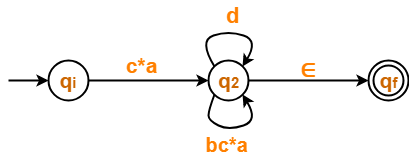### Step-04:

Now, let us eliminate state q2.

• There is a path going from state qi to state qf via state q2 .
• So, after eliminating state q2, we put a direct path from state qi to state qf having cost c*a(d+bc*a)*∈ = c*a(d+bc*a)*

Eliminating state q2, we get-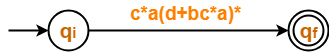From here,

 Regular Expression = c*a(d+bc*a)*

## Problem-04:

Find regular expression for the following DFA-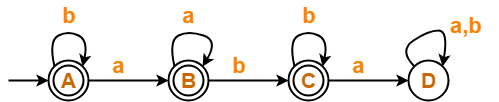## Solution-

### Step-01:

• State D is a dead state as it does not reach to any final state.
• So, we eliminate state D and its associated edges.

The resulting DFA is-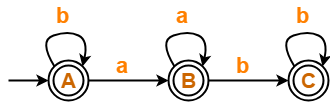### Step-02:

• Initial state A has an incoming edge (self loop).
• So, we create a new initial state qi.

The resulting DFA is-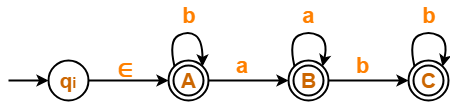### Step-03:

• There exist multiple final states.
• So, we convert them into a single final state.

The resulting DFA is-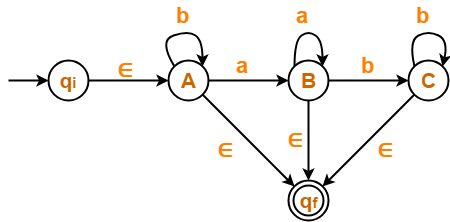### Step-04:

Now, we start eliminating the intermediate states.

First, let us eliminate state C.

• There is a path going from state B to state qf via state C.
• So, after eliminating state C, we put a direct path from state B to state qf having cost b.b*.∈ = bb*

Eliminating state C, we get-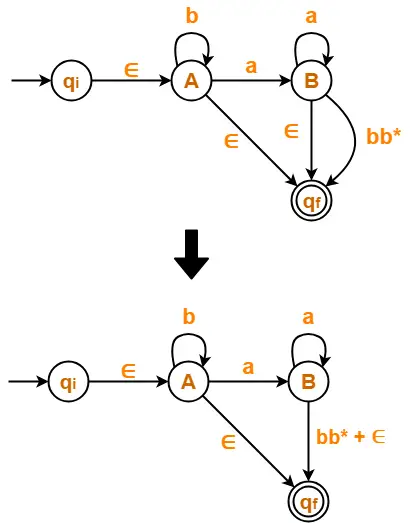### Step-05:

Now, let us eliminate state B.

• There is a path going from state A to state qf via state B.
• So, after eliminating state B, we put a direct path from state A to state qf having cost a.a*.(bb*+∈) = aa*(bb*+∈)

Eliminating state B, we get-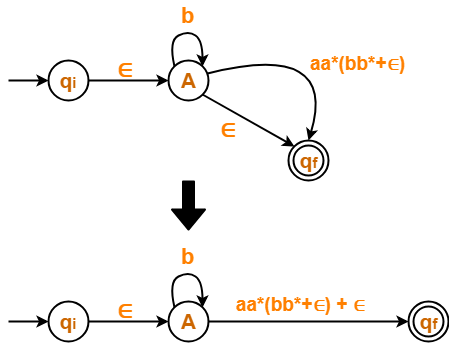### Step-06:

Now, let us eliminate state A.

• There is a path going from state qi to state qf via state A.
• So, after eliminating state A, we put a direct path from state qi to state qf having cost ∈.b*.(aa*(bb*+∈)+∈) = b*(aa*(bb*+∈)+∈)

Eliminating state A, we get-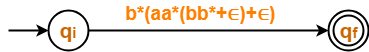From here,

 Regular Expression = b*(aa*(bb*+∈)+∈)

We know, bb* + ∈ = b*

So, we can also write-

 Regular Expression = b*(aa*b*+∈)

## Problem-05:

Find regular expression for the following DFA-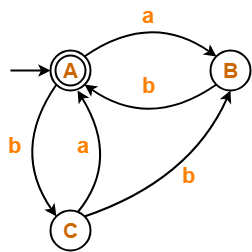## Solution-

### Step-01:

• Since initial state A has an incoming edge, so we create a new initial state qi.
• Since final state A has an outgoing edge, so we create a new final state qf.

The resulting DFA is-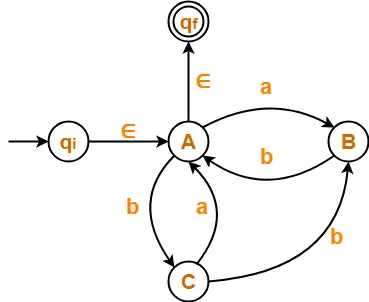### Step-02:

Now, we start eliminating the intermediate states.

First, let us eliminate state B.

• There is a path going from state C to state A via state B.
• So, after eliminating state B, we put a direct path from state C to state A having cost b.b = bb.
• There is a loop on state A using state B.
• So, after eliminating state B, we put a direct loop on state A having cost a.b = ab.

Eliminating state B, we get-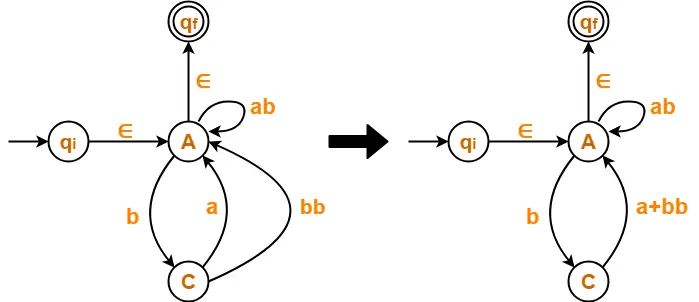### Step-03:

Now, let us eliminate state C.

• There is a loop on state A using state C.
• So, after eliminating state C, we put a direct loop on state A having cost b.(a+bb) = b(a+bb)

Eliminating state C, we get-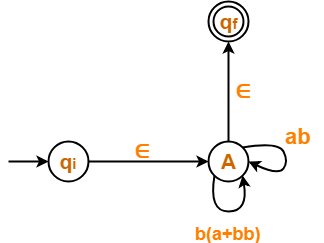### Step-04:

Now, let us eliminate state A.

• There is a path going from state qi to state qf via state A.
• So, after eliminating state A, we put a direct path from state qi to state qf having cost ∈.(ab + b(a+bb))*∈ = (ab + b(a+bb))*

Eliminating state A, we get-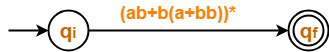From here,

 Regular Expression = (ab + b(a+bb))*

## Problem-06:

Find regular expression for the following DFA-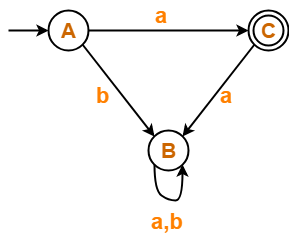## Solution-

• State B is a dead state as it does not reach to the final state.
• So, we eliminate state B and its associated edges.

The resulting DFA is-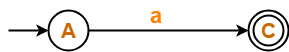From here,

 Regular Expression = a

## Problem-07:

Find regular expression for the following DFA-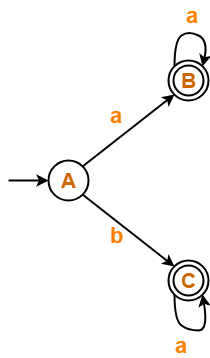## Solution-

### Step-01:

• There exist multiple final states.
• So, we create a new single final state.

The resulting DFA is-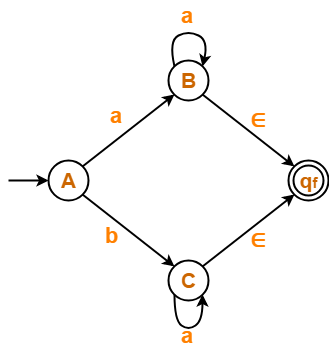### Step-02:

Now, we start eliminating the intermediate states.

First, let us eliminate state B.

• There is a path going from state A to state qf via state B.
• So, after eliminating state B, we put a direct path from state A to state qf having cost a.a*.∈ = aa*.

Eliminating state B, we get-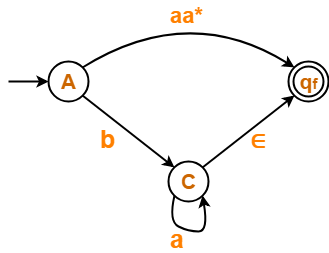### Step-03:

Now, let us eliminate state C.

• There is a path going from state A to state qf via state C.
• So, after eliminating state C, we put a direct path from state A to state qf having cost b.a*.∈ = ba*.

Eliminating state C, we get-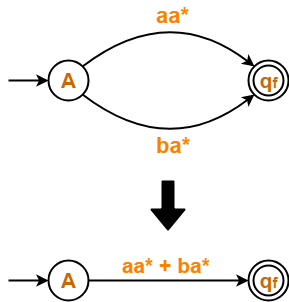From here,

 Regular Expression = aa* + ba*

To gain better understanding about Converting DFA to Regular Expression,

Watch this Video Lecture

Next Article- Arden’s Theorem

Get more notes and other study material of Theory of Automata and Computation.

Watch video lectures by visiting our YouTube channel LearnVidFun.